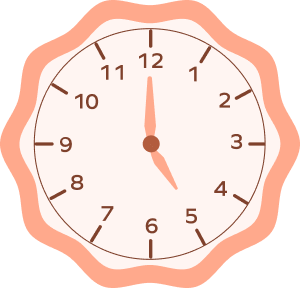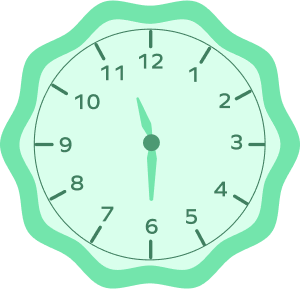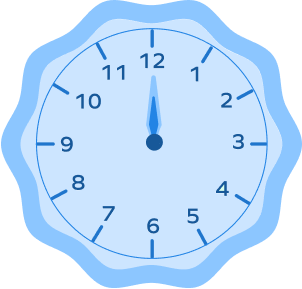# Hour Hand On a Clock

Do you know what a clock is? A tool used to measure and tell time is called a clock or a timepiece.

In our everyday life, we see two different types of clocks: Analog Clock and Digital Clock.

## What is an Analog Clock?

Analog clock is a type of a clock in which the hours, minutes, and sometimes seconds are indicated by hands on a dial.

## What is a Digital Clock?

Digital clock displays the time in numerical digits or numbers and not by hands on a dial.

## What is the Hour Hand on a Clock?

There are three hands in a clock, the hour hand, the minute hand, and the second hand. The smallest one is the hour hand and the hand bigger than the hour hand is the minute hand. The second hand moves relatively quicker than the other two hands. The hour hand on a clock represents the hour. On an analog clock, you look in the direction the hands are pointing to read the time. The shortest hand or the little hand displays the current hour, the longest or big hand displays the current minute, and the thinnest hand displays the current second. In a day, there are 24 hours, and since the clock only has numbers till 12, the hour hand goes around the clock 2 times. Once, from midnight to noon and then noon to midnight.

## What Defines an Hour on a Clock?

The space between two numbers on the clock represents an hour. When the hour hand on a clock travels from one number to the next, it represents the passing of one hour. The minute hand crosses the whole round of the clock from twelve to twelve because the hour hand goes slowly.

## Reading Time Using the Hour Hand

Let’s take an example: the minute hand is at 12, and the hour hand is at 1. This means that the hour of the number 1 has started. And we’ll call this time 1 o’clock.

In the hour of the number 1, the minute hand takes a whole round and passes through each number from 12 to 12. When it is at 6, the minute hand has traveled half a round of the clock and the hour hand also comes halfway between 1 and 2, and we say that half an hour has passed.

As the minute hand moves and takes a whole round, we say that the hour of 1 has passed. And then, when the hour hand on a clock reaches 2 and minute hand takes a round and reaches 12, we say that the hour of the number 2 has started and we call this time 2 o’clock.

After two hours, the hour hand would go to 4, and the minute hand would also have completed two rounds of the clock. That time will be 4 o’clock.

## Facts about Hour Hand on a Clock

• It takes one hour to move from one big division to the next.
• It takes 12 hours to complete one round.
• Every hour, both hands coincide once. They will coincide 11 times in 12 hours. It occurs due to a single incident between 12 and 1 o’clock.
• When the hands coincide or oppose each other, they are in the same straight line.
• When the hands are facing each other, they are 30 minutes apart.
• If the hour and minute hands move at their usual speeds, they will meet after 65 minutes.

## Solved Examples

Example 1: What is the time when the hour hand points at 5 and the minute hand points at 12?

Solution: 5 o’clock.

Explanation:

When the hour hand is at any number and the minute hand is at 12, it marks the beginning of that hour. If the hour hand is at 5, and the minute hand is at 12, we call it the hour of 5 or 5 o’clock.

Example 2: What is the time when the hour hand is halfway between 5 and 6 and the minute hand is pointing at 6?

Solution: Half past 5

Explanation:

When the hour hand is halfway between 5 and 6, it means half an hour has passed after 5 o’clock, and we call that “half past 5.”

Example 3: Which is the slowest hand in the clock?Solution: The hour hand is the slowest hand on the clock.

## Practice Problems

1

### What would be the time shown by the given clock after three hours?7 o’clock
8 o’clock
9 o’clock
10 o’clock
CorrectIncorrect
After three hours, the hour hand will move 3 numbers forward and come to 8, and the minute hand will be at 12. The time will be 8 o’clock.
2

### What is the time shown on the clock?Half past 6
Half past 11
6 o’clock
11 o’clock
CorrectIncorrect
Correct answer is: Half past 11
The hour hand is halfway between 11 and 12, this means half an hour has passed after 11 o’clock, and we call that half past 11.
3

### How many hours will it take for the hour hand to go around the clock in a day?

24 hours
1 hour
12 hours
60 minutes
CorrectIncorrect